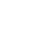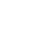LV. 4
GP 109# 【攻略】抽包的數學（未完成）（更新提前重置與否的比較）

GP34 BP-

（雖然每包是3張，但SR以上每次最多一張，也就是不會出現一包同時有兩張想要的卡的情況。）

Python程序

# dl.py

def dld(n, x, y):
if x * y == 0:
return [(x + y) * (n + 1) / 2., 0., 0.]
else:
s = 0
fx = dld(n, x - 1, y)
fy = dld(n, x, y - 1)
fxy = dld(n, x - 1, y - 1)
stopx = 2 * fxy - 2 * fx + n + 1
stopy = 2 * fxy - 2 * fy + n + 1

for m in range(1, n):
ex = fx if m <= stopx else fxy + (n + 1 - m) / 2.
ey = fy if m <= stopy else fxy + (n + 1 - m) / 2.
s += (ex + ey + 2 * m) * (n - m) / (n * n - n)
return [s, stopx, stopy]

def dlc(x, y):
if x * y == 0:
return [(x + y) / 2., 0., 0.]
else:
fx = dlc(x - 1, y)
fy = dlc(x, y - 1)
fxy = dlc(x - 1, y - 1)

return [-2 * (fx ** 3 + fy ** 3) / 3. + 2 * (fx ** 2 + fy ** 2) * fxy - 2 * (fx + fy) * fxy ** 2 + (fx + fy) / 2. + 4 * fxy ** 3 / 3. + 1 / 3., 2 * fxy - 2 * fx + 1., 2 * fxy - 2 * fy + 1.] # last two are stopx and stopy

>>> import dl
>>> dl.dld(100, 1, 1)
[67.33333333333336, 0.0, 0.0]
>>> dl.dld(100, 1, 2)
[109.12333333333335, 0.0, 67.33333333333329]
>>> dl.dld(100, 2, 2)
[133.3097232323233, 17.420000000000016, 17.420000000000016]

>>> import dl
>>> dl.dlc(1, 1)
[0.6666666666666667, 0.0, 0.0]
>>> dl.dlc(1, 2)
[1.080246913580247, 0.0, 0.6666666666666665]
>>> dl.dlc(2, 2)
[1.3192571392371564, 0.17283950617283939, 0.17283950617283939]

 100 1 2 3 1 (67.33, 0.00, 0.00) (109.12, 0.00, 67.33) (155.53, 0.00, 84.75) 2 (133.31, 17.42, 17.42) (170.68, 8.18, 52.63) 3 (197.60, 26.26, 26.26)

 80 1 2 3 1 (54.00, 0.00, 0.00) (87.52, 0.00, 54.0) (124.74, 0.00, 67.96) 2 (106.93, 13.96, 13.96) (136.90, 6.56, 42.18) 3 (158.50, 21.06, 21.06)

 200 1 2 3 1 (140, 0.00, 0.00) (217.15, 0.00, 134.00) (309.51, 0.00, 168.70) 2 (339.58, 34.70, 34.70) (339.58, 16.28, 104.83) 3 (393.09, 52.30, 52.30)

 180 1 2 3 1 (120.67, 0.00, 0.00) (195.54, 0.00, 120.67) (278.71, 0.00, 151.91) 2 (238.85, 31.25, 31.25) (305.80, 14.66, 94.39) 3 (353.99, 47.10, 47.10)

------------------------------------------------------

100包的卡，需要獨有A卡3張、B卡3張、C卡2張、D卡1張、E卡1張。先換個角度去看所要的卡：

101*(5/6 + 3/4 + 2/3) = 227.25

 100 1 2 3 1 (67.33, 67.33) (109.12, 117.83) (155.53, 168.33) 2 (133.31, 134.67) (170.68, 185.17) 3 (197.60, 202.00)

face基於日前微軟官方表示 Internet Explorer 不再支援新的網路標準，可能無法使用新的應用程式來呈現網站內容，在瀏覽器支援度及網站安全性的雙重考量下，為了讓巴友們有更好的使用體驗，巴哈姆特即將於 2019年9月2日 停止支援 Internet Explorer 瀏覽器的頁面呈現和功能。